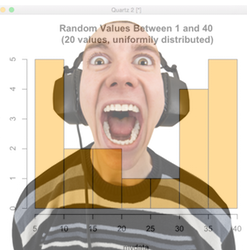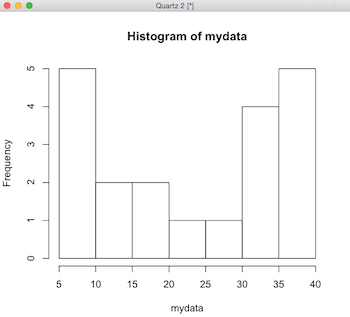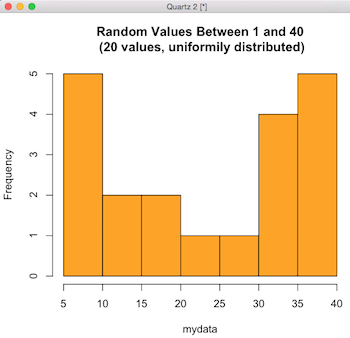## How To Create Histograms Using The Free Statistics Package R

Posted on 12-May-2015 by Craig Shallahamer, craig@orapub.comI'm willing to bet your million dollar IO subsystem is returning some Oracle single block reads in over one second.

Not one millisecond or 100 milliseconds but more like 1000 milliseconds. And guess what?

This is normal behavior for most production Oracle Database IO subsystems. Why? Because super high concurrency happens and sometimes it is really bad... but just not that often.

So, how do you know the true situation in your Oracle system? For starters, we need to create and understand histograms. Not Oracle histograms, but statistical histograms. That's what this posting is all about.

In my last post I focused on how to install R. If you have not installed R yet, you will find it is super simple and fast!

### How To Create A Histogram In R

Let's start by generating and then displaying 20 samples between 1 and 40. Here is one way to do this in R.

``````> mydata <- sample(1:40, 20, replace=T)
> mydata
 27 37 11 34 36 36  7  7 19  6  9 40 39 20 31 35 24  7
 35 11
``````

Now let's create a histogram based on our data so we can better understand our data by seeing it in a non numerical way. Here is one way to do this in R.

``````> hist(mydata)
``````Simple, eh? But I hear you. It looks sterile and academic. Here's how to create a sweeeeet looking histogram that you can be proud of.

``````> var1="(20 values, uniformly distributed)"
> hist(mydata,col="orange",breaks=11,main=paste("Random Values Between 1 and 40\n",var1))
``````I threw in four cool R tricks that I use to create flexible and appealing histograms. First, I set the color to orange. Second, I set a variable, named var1. Third, I inserted the variable into the title. And finally, I used the breaks=11 command.

The breaks command encourages R to use a specified number of bins when creating the histogram. But as you can see, it ignored my 11 bin request. More than the default number of bins can be very useful when I want to see more details near the smaller values. Think: Oracle wait times less than 50ms.

Visualizing data is great, but we also need to see a numeric summary of our data set... the statistics.

### How To Generate Basic Statistics In R

Statistics are numeric summaries of our data. This can be helpful when we have more than a couple sample values. Here's how to calculate the mean, median and standard deviation (which measures how disperse our samples are).

``````> summary(mydata)
Min. 1st Qu.  Median    Mean 3rd Qu.    Max.
6.00   10.50   25.50   23.55   35.25   40.00
> sd(mydata)
 12.83283
``````

### Have You Ever Heard, "Sixty-Eight Percent..."

If our sample set is normally distributed, then 68% of our samples are between minus one standard deviation and plus one standard deviation. For our data set, that means about 14 of our samples (68% X 20 samples) are between 10.72 (23.55-12.83) and 36.38 (23.55+12.83).

I couldn't resist, so I checked our sample data. And, guess what? There are 13 samples within this range. Not bad. So, our data is normally distributed, right? Wrong. Read on...

### How To Test If Your Data Is Normal

To check if our data is normal, we need to perform a statistical test. Surprise, it's called a normality test. (I think I was given a few of these tests over the years...) There are many accepted normality tests, such as the Shapiro-Wilk. Here's how to do the test in R.

``````> shapiro.test(mydata)

Shapiro-Wilk normality test

data:  mydata
W = 0.86, p-value = 0.007863
``````

We want a p-value greater than 0.05. Since the p-value is 0.0079, our data is not likely normally distributed. Looking at our orange histogram above, it should not be a surprise that our data is not normally distributed.

### Why Do A Normality Test? (IMPORTANT)

If your data is not confirmed normal, then never say 68% of your data is between plus/minus one standard deviation or that 95% of your data is between plus/minus two standard deviations. Only if our sample data is normal or we checked the data ourselves (like I did), can we make such a sweeping claim.

People make this misleading mistake all the time. Especially MBA types and those who assume that every set of data is normally distributed. ...but I digress.

If you want to see what the histogram and statistics look like for a normally distributed sample set, try the following R command to generate your sample set.

``````> mydata=rnorm(200, mean=12, sd=1.2)
``````

Play around with the number of samples. You will be amazed at how many samples it takes before you can visually tell the sample set is likely normal. If you want more bins displayed when creating the histogram, don't forget to use the breaks= option.

### What's Next?

At this point you're familiar with R, basic statistics and histograms. Now it's time to bring Oracle performance into the mix.

Specifically, this means bringing Oracle wait event times into the mix! The db file sequential read and log file sync wait events are especially helpful in understanding your IO subsystem's performance. How to do this and more will be the topic of my next article.